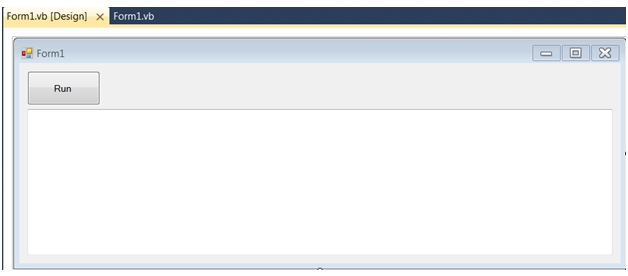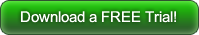## XLeratorDLL vs WorksheetFunction: YIELD

Aug 21

Written by: Charles Flock
8/21/2016 3:28 PMIn this article we compare the XLeratorDLL/financial YIELD function to Worksheetfunction YIELD where we encounter a few surprises and some things that made me scratch my head.
The calculation of yield is an essential part of the math associated with the pricing of a bond. Given the yield, you can calculate the price of the bond for any settlement date. Conversely, given the price you should be able to calculate the yield for a settlement date. The yield calculation is much more involved than the price calculation since there is no closed-form solution if the settlement occurs before the last coupon period. And the YIELD function in Excel works fine, though it has limitations. The cause for head-scratching is that it is not a member of Worksheetfunction.
I have the following statements at the top of my code.
Option Explicit On
Option Strict On

Imports System.Runtime.InteropServices
Imports System.Text
Imports Excel = Microsoft.Office.Interop.Excel
Imports wct = XLeratorDLL_financial.XLeratorDLL_financial
Then I create a very simple form which consists of a single button and a text box where the results are displayed.Let's look at how the YIELD function works in XLDLL.
Public Shared Sub testYIELD()
Dim Settlement As Date = #5/1/2014#    'Settlement Date: 2034-06-015
Dim Maturity As Date = #6/15/2034#     'Maturity Date: 2014-05-01
Dim Rate As Double = 0.025             'Coupon Rate: 2.50%
Dim Price As Double = 96.0             'Price: 96.00
Dim Redemption As Double = 100         'Redemption: 100
Dim Frequency As Double = 2            'Frequency: twice-yearly
Dim Basis As String = "1"              'Basis: Actual/Actual
Dim result As Double

result = wct.YIELD(Settlement, Maturity, Rate, Price, Redemption, Frequency, Basis)

Form1.TextBox1.AppendText("XLDLL YIELD: " & result.ToString("F8"))

End Sub
You would expect the code for the Excel function to be pretty much the same, as it was with the XIRR function. This is what I coded at first.
Public Shared Sub testYIELD()
Dim XL As New Excel.Application
Dim Settlement As Date = #5/1/2014#    'Settlement Date: 2034-06-015
Dim Maturity As Date = #6/15/2034#     'Maturity Date: 2014-05-01
Dim Rate As Double = 0.025             'Coupon Rate: 2.50%
Dim Price As Double = 96.0             'Price: 96.00
Dim Redemption As Double = 100         'Redemption: 100
Dim Frequency As Double = 2            'Frequency: twice-yearly
Dim Basis As String = "1"              'Basis: Actual/Actual
Dim result As Double

result = XL.WorksheetFunction.YIELD(Settlement, Maturity, Rate, Price, Redemption, Frequency, Basis)

Form1.TextBox1.AppendText("XLDLL YIELD: " & result.ToString("F8") & vbCrLf)

XL.Quit()
End Sub
But that code won't run because YIELD is not a method in Worksheetfunction. After digging around a little bit, I come to the conclusion that if I use the Evaluate function and construct a properly formatted string, I might be able to get a result.
Public Shared Sub testYIELD()
Dim XL As New Excel.Application
Dim Settlement As Date = #5/1/2014#    'Settlement Date: 2034-06-015
Dim Maturity As Date = #6/15/2034#     'Maturity Date: 2014-05-01
Dim Rate As Double = 0.025             'Coupon Rate: 2.50%
Dim Price As Double = 96.0             'Price: 96.00
Dim Redemption As Double = 100         'Redemption: 100
Dim Frequency As Double = 2            'Frequency: twice-yearly
Dim Basis As String = "1"              'Basis: Actual/Actual
Dim strYield As String                 'Used to concatenate input parameters
Dim result As Double

'result = XL.WorksheetFunction.YIELD(Settlement, Maturity, Rate, Price, Redemption, Frequency, Basis)

strYield = "YIELD(" & Settlement.ToOADate.ToString & "," & Maturity.ToOADate.ToString & "," & Rate.ToString & "," & _
Price.ToString & "," & Redemption.ToString & "," & Frequency.ToString & "," & Basis & ")"

result = Convert.ToDouble(XL.Evaluate(strYield))

Form1.TextBox1.AppendText("Excel YIELD: " & result.ToString)

XL.Quit()
End Sub
I add the following code to Button1_Click to produce the results of the comparison.
Private Sub Button1_Click(sender As System.Object, e As System.EventArgs) Handles Run.Click
TextBox1.Clear()
test_XLDLL.testYIELD()
test_XL.testYIELD()
End Sub
When I run the code I get the following result.
In order to evaluate the relative performance we need to incorporate the overhead of constructing a new string every time the Excel version is invoked. In the interest of keeping things relatively simple, this code will randomly generate 10,000 values for Settlement, Maturity, Rate, and Price while holding Frequency, Redemption and Basis constant. We then build a new string every time the function is invoked.
Public Shared Sub testYIELD()
Dim XL As New Excel.Application
Dim Settlement As Date = #5/1/2014#   'Settlement Date: 2034-06-015
Dim Redemption As Double = 100        'Redemption: 100
Dim Frequency As Double = 2           'Frequency: twice-yearly
Dim Basis As String = "1"             'Basis: Actual/Actual
Dim strYield As String                'Used to concatenate input parameters
Dim result As Double
Dim r1 As New System.Random
Dim r2 As New System.Random
Dim S As Date()
Dim M As Date()
Dim R As Double()
Dim P As Double()
Dim n As Integer = 10000

ReDim S(n)
ReDim M(n)
ReDim R(n)
ReDim P(n)

For i As Integer = 1 To n
R(i) = Math.Round(0.000001 + r2.NextDouble * 0.08, 6)
P(i) = Math.Round(96 + r2.NextDouble * 8, 6)
Next

Dim time_start As Date
Dim time_end As Date

time_start = Now
For i As Integer = 1 To n
strYield = "YIELD(" & S(i).ToOADate.ToString & "," & M(i).ToOADate.ToString & "," & R(i).ToString & "," & _
P(i).ToString & "," & Redemption.ToString & "," & Frequency.ToString & "," & Basis & ")"
result = Convert.ToDouble(XL.Evaluate(strYield))
Next
time_end = Now

Form1.TextBox1.AppendText("Excel YIELD elapsed time: " & (time_end - time_start).TotalMilliseconds.ToString("F4") & " milliseconds" & vbCrLf)

XL.Quit()
End Sub
And we do the same thing for the XLDLL version.
Public Shared Sub testYIELD()
Dim Settlement As Date = #5/1/2014#   'Settlement Date: 2034-06-015
Dim Redemption As Double = 100        'Redemption: 100
Dim Frequency As Double = 2           'Frequency: twice-yearly
Dim Basis As String = "1"             'Basis: Actual/Actual
Dim result As Double
Dim r1 As New System.Random
Dim r2 As New System.Random
Dim S As Date()
Dim M As Date()
Dim R As Double()
Dim P As Double()
Dim n As Integer = 10000

ReDim S(n)
ReDim M(n)
ReDim R(n)
ReDim P(n)

For i As Integer = 1 To n
R(i) = Math.Round(0.000001 + r2.NextDouble * 0.08, 6)
P(i) = Math.Round(96 + r2.NextDouble * 8, 6)
Next

Dim time_start As Date
Dim time_end As Date

time_start = Now
For i As Integer = 1 To n
result = wct.YIELD(S(i), M(i), R(i), P(i), Redemption, Frequency, Basis)
Next
time_end = Now

Form1.TextBox1.AppendText("XLDLL YIELD elapsed time: " & (time_end - time_start).TotalMilliseconds.ToString("F4") & " milliseconds" & vbCrLf)

End Sub
When the code is executed, I get the following result.
The XLDLL function is slightly more than 19 times faster than using the Excel version.
In terms of industry practice the Excel YIELD function also has some problems, most notably that it does not accept negative values for rate (the Bank of Japan, the European Central Bank, and several European agencies now have negative rates). In Excel, you will get NUM#! in the cell when you try this. Let's see what happens.
In the XLDLL code:
Public Shared Sub testYIELD()
result = wct.YIELD(Today, Today.AddDays(365), -0.0025, 101.5, 100, 2, "1")
Form1.TextBox1.AppendText("XLDLL YIELD: " & result.ToString("F8") & vbCrLf)
End Sub
In the Excel code:
Public Shared Sub testYIELD()
Dim XL As New Excel.Application
Try
Catch ex As Exception
result = Double.NaN
End Try
Form1.TextBox1.AppendText("Excel YIELD: " & result.ToString("F8") & vbCrLf)
XL.Quit()
End Sub
This returns the following result.
What's up with the Excel calculation? After a little bit of digging it turns out that that -2146826252 is actually equivalent to NUM#! in the COM world. This means that Try…Catch is not going to work and if we were going to do this in Excel, we would need to incorporate something like this.
Public Shared Sub testYIELD()
Dim XL As New Excel.Application
Try
Catch ex As Exception
result = Double.NaN
End Try

Select Case result
Case -2146826281        '#DIV/0!
result = Double.NaN
Case -2146826246        '#N/A!
result = Double.NaN
Case -2146826259        '#NAME!
result = Double.NaN
Case -2146826288        '#NULL
result = Double.NaN
Case -2146826252        '#NUM!
result = Double.NaN
Case -2146826265        '#REF
result = Double.NaN
Case -2146826273        '#VALUE!
result = Double.NaN
End Select

Form1.TextBox1.AppendText("Excel YIELD: " & result.ToString("F8") & vbCrLf)
XL.Quit()
End Sub
That give us the following result, which shows that Excel cannot handle the negative rate.
It also turns out that the Worksheetfunction PRICE method doesn't handle negative rates and, more problematically, doesn't handle negative yields. This XLDLL code demonstrates how things should work.
Public Shared Sub testYIELD()
Dim y As Double
Dim p As Double

y = wct.YIELD(Today, Today.AddDays(365), 0.0025, 101, 100, 2, "1")
p = wct.PRICE(Today, Today.AddDays(365), 0.0025, y, 100, 2, "1")

Form1.TextBox1.AppendText("XLDLL YIELD: " & y.ToString("F8") & vbCrLf)
Form1.TextBox1.AppendText("XLDLL PRICE: " & p.ToString("F8") & vbCrLf)
End Sub
And we can put the same code in our Excel test.
Public Shared Sub testYIELD()
Dim XL As New Excel.Application
Dim y As Double
Dim p As Double

Try
p = XL.WorksheetFunction.Price(Today, Today.AddDays(365), 0.0025, y, 100, 2, 1)
Catch ex As Exception
p = Double.NaN
End Try

Form1.TextBox1.AppendText("Excel YIELD: " & y.ToString("F8") & vbCrLf)
Form1.TextBox1.AppendText("Excel PRICE: " & p.ToString("F8") & vbCrLf)

XL.Quit()
End Sub
This produces the following result.
In addition to these aforementioned problems, the Excel YIELD does not handle monthly coupons, or coupons every 28 days, has no provision for end of month rules (where a bonds pays it's coupons on the 30th of the month not the last day of the month), and only supports a few day count conventions (as opposed to the 2 dozen in XLeratorDLL and Bloomberg). There is also no built-in capability to calculate the dirty price of a bond, which is needed to calculate the settlement amount of the bond, and there is no way to calculate ex-dividend bonds all of which can be accommodated in XLDLL.
If you are interested in having a 21st century bond figuration capability in your .NET code, you should try the free 15-day trial of XLeratorDLL/financial. It takes about a minute to download and about a minute to install and comes with loads of C# and VB.NET examples that you can use as templates to start coding.
All the documentation is available on-line at westclintech.com. If you have any questions or there is something that you would like to see added, just send an e-mail to support@westclintech.com.Tags:
Categories:
Location: BlogsThe WestClinTech Blog

## Search Blogs

 KeywordsPhrase

## Blog Archives

Archive
 < December 2023 >
SunMonTueWedThuFriSat
262728293012
3456789
10111213141516
17181920212223
24252627282930
31123456
Monthly
Go

### SupportCopyright 2008-2023 Westclintech LLC         Privacy Policy        Terms of Service